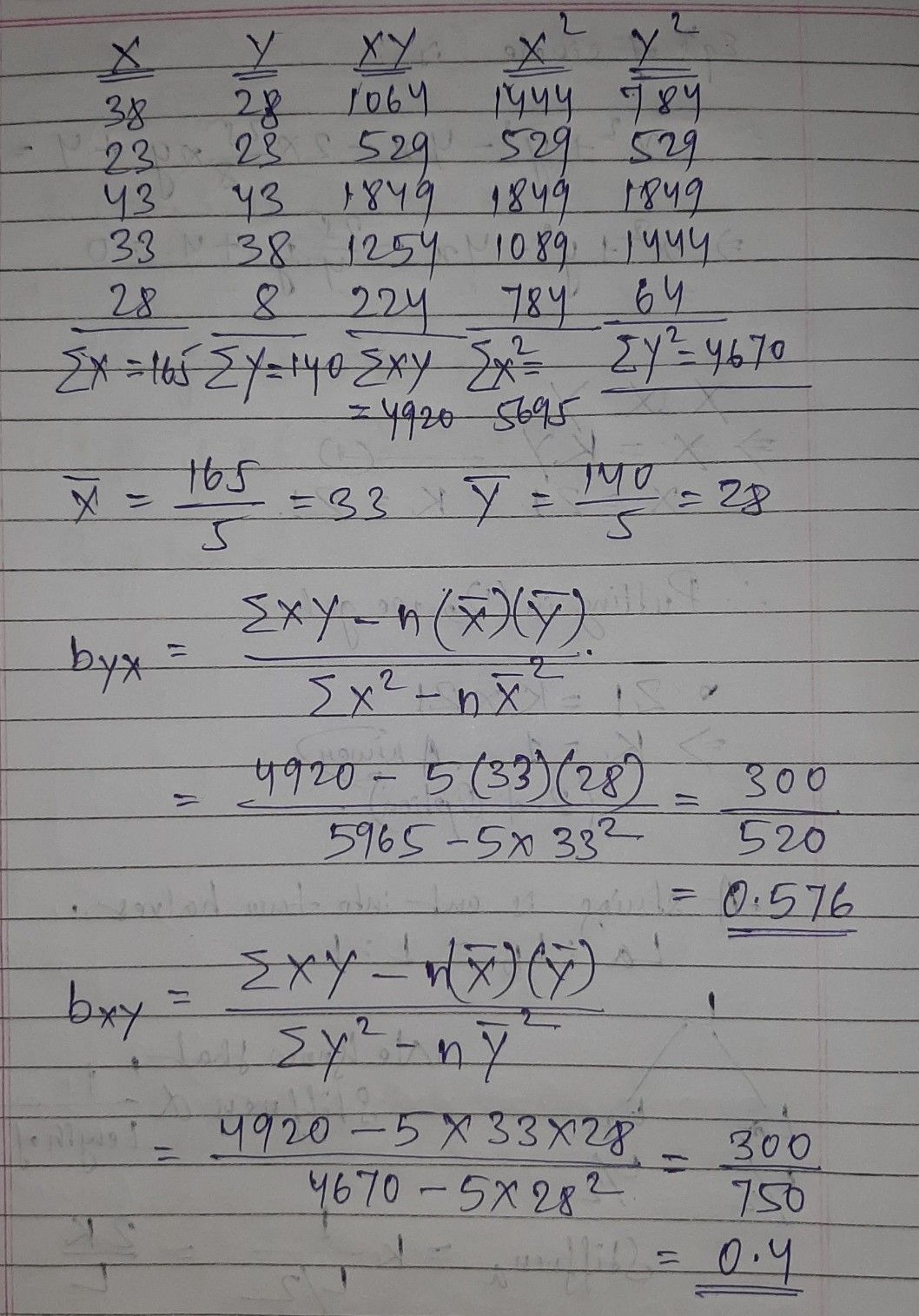Symbol
Problem$92=$ WITHOUT TAKING ANY DEVIATION 4) The two regression coefficients for the following data are (€) Y $38$ $28$ $23$ $23$ $43$ $43$ $33$ $38$ $28$ 8 a) $12$ and $0.4$ b) $1.6$ and $0.8$ c) $1.7$ and $0.8$ d) $1.8$ and $0.3$
10th-13th grade
Probability and Statistics
Search count: 106
SolutionQanda teacher - Bhanu